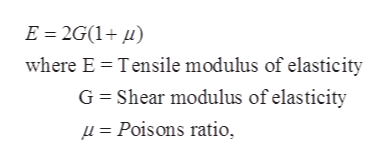# in metallics, the shear modulus of elasticity G related to the tensile modulus E, on average, by?a) G=70% of E, on averageb) G= 30% of E, on averagec) 50% of E, on averaged) 40% of E, on average

Question

in metallics, the shear modulus of elasticity G related to the tensile modulus E, on average, by?

a) G=70% of E, on average

b) G= 30% of E, on average

c) 50% of E, on average

d) 40% of E, on average

check_circle

Step 1

The relationship between E and G for the metallics material is given as the-help_outlineImage TranscriptioncloseE 2G(1 where E Tensile modulus of elasticity G Shear modulus of elasticity Poisons ratio fullscreen
Step 2

For the metallic materials the average value of poisons ratio is

0 < π < 0.5

The Average of poison ratio is

Step 3

Put the average value of the poisons ra...

### Want to see the full answer?

See Solution

#### Want to see this answer and more?

Solutions are written by subject experts who are available 24/7. Questions are typically answered within 1 hour.*

See Solution
*Response times may vary by subject and question.
Tagged in

### Classical Mechanics